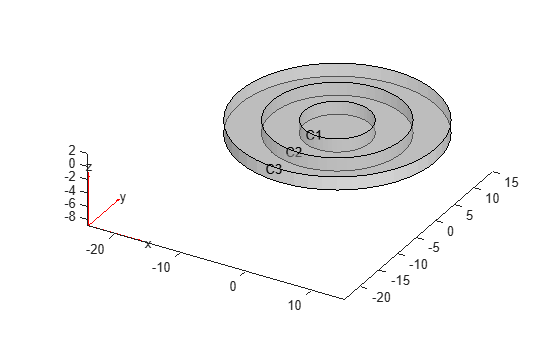# findStructuralIC

Find initial displacement and velocity assigned to geometric region

## Syntax

``sica = findStructuralIC(structuralmodel.InitialConditions,RegionType,RegionID)``

## Description

example

````sica = findStructuralIC(structuralmodel.InitialConditions,RegionType,RegionID)` returns the initial displacement and velocity assigned to the specified region.```

## Examples

collapse all

Find the initial displacement and velocity assigned to the cells of a 3-D geometry.

Create a transient dynamic model for a 3-D problem.

`structuralmodel = createpde("structural","transient-solid");`

Create the geometry consisting of the three nested cylinders and include it in the model. Plot the geometry.

```gm = multicylinder([5 10 15],2); structuralmodel = createpde("structural","transient-solid"); structuralmodel.Geometry = gm; pdegplot(structuralmodel,"CellLabels","on","FaceAlpha",0.5)```Set the initial conditions for each cell. When you specify only the initial velocity or initial displacement, `structuralIC` assumes that the omitted parameter is zero.

```structuralIC(structuralmodel,"Displacement",[0;0;0],... "Velocity",[0;0;0], ... "Cell",1); structuralIC(structuralmodel,"Displacement",[0;0.1;0], ... "Cell",2); structuralIC(structuralmodel,"Velocity",[0;0.2;0], ... "Cell",3);```

Check the initial condition specification for cell 1.

`SICACell1 = findStructuralIC(structuralmodel.InitialConditions,"Cell",1)`
```SICACell1 = GeometricStructuralICs with properties: RegionType: 'Cell' RegionID: 1 InitialDisplacement: [3x1 double] InitialVelocity: [3x1 double] ```
`SICACell1.InitialDisplacement`
```ans = 3×1 0 0 0 ```
`SICACell1.InitialVelocity`
```ans = 3×1 0 0 0 ```

Use a static solution as an initial condition for a dynamic structural model. Check and plot the initial displacement.

Create a static model.

`staticmodel = createpde("structural","static-solid");`

Create the geometry and include it in the model. Plot the geometry.

```gm = multicuboid(0.06,0.005,0.01); staticmodel.Geometry = gm; pdegplot(staticmodel,"FaceLabels","on","FaceAlpha",0.5) view(50,20)```Specify Young's modulus, Poisson's ratio, and the mass density.

```structuralProperties(staticmodel,"YoungsModulus",210E9, ... "PoissonsRatio",0.3,... "MassDensity",7800);```

Apply the boundary condition and static load.

```structuralBC(staticmodel,"Face",5,"Constraint","fixed"); structuralBoundaryLoad(staticmodel,"Face",3, ... "SurfaceTraction",[0;1E6;0]); generateMesh(staticmodel,"Hmax",0.02); Rstatic = solve(staticmodel);```

Create a dynamic model and assign geometry.

```dynamicmodel = createpde("structural","transient-solid"); gm = multicuboid(0.06,0.005,0.01); dynamicmodel.Geometry = gm;```

Apply the boundary condition.

`structuralBC(dynamicmodel,"Face",5,"Constraint","fixed");`

Specify the initial condition using the static solution.

```generateMesh(dynamicmodel,"Hmax",0.02); structuralIC(dynamicmodel,Rstatic)```
```ans = NodalStructuralICs with properties: InitialDisplacement: [113x3 double] InitialVelocity: [113x3 double] ```

Check the initial condition specification for `dynamicmodel`.

`sica = findStructuralIC(dynamicmodel.InitialConditions,"Cell",1)`
```sica = NodalStructuralICs with properties: InitialDisplacement: [113x3 double] InitialVelocity: [113x3 double] ```

Plot the `z`-component of the initial displacement.

```pdeplot3D(dynamicmodel,"ColorMapData",sica.InitialDisplacement(:,3)) title("Initial Displacement in the Z-direction")```## Input Arguments

collapse all

Initial conditions of a transient structural model, specified as the `InitialConditions` property of a `StructuralModel` object.

Geometric region type, specified as `"Face"`, `"Edge"`, or `"Vertex"` for a 2-D model or 3-D model, or `"Cell"` for a 3-D model.

Data Types: `char`

Geometric region ID, specified as a vector of positive integers. Find the region IDs by using `pdegplot`.

Data Types: `double`

## Output Arguments

collapse all

Structural initial condition for a particular region, returned as a `GeometricStructuralICs` or `NodalStructuralICs` object. For details, see GeometricStructuralICs Properties and NodalStructuralICs Properties.

## Version History

Introduced in R2018a